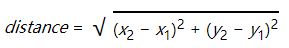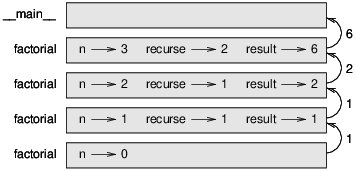# # 第六章 有返回值的函数

## # 6.1 返回值

``````    e = math.exp(1.0)
``````
1
2

``````    def area(radius):
return a
``````
1
2
3

``````    def area(radius):
``````
1
2

``````    def absolute_value(x):
if x < 0:
return -x
else:
return x
``````
1
2
3
4
5

``````    def absolute_value(x):
if x < 0:
return -x
if x > 0:
return x
``````
1
2
3
4
5

``````    >>> absolute_value(0)
>>> absolute_value(0)
None
``````
1
2
3

## # 6.2 增量式开发``````    def distance(x1, y1, x2, y2):
return 0.0
``````
1
2

``````    >>> distance(1, 2, 4, 6)
0.0
``````
1
2

``````    def distance(x1, y1, x2, y2):
dx = x2 - x1
dy = y2 - y1
print('dx is', dx)
print('dy is', dy)
return 0.0
``````
1
2
3
4
5
6

``````    def distance(x1, y1, x2, y2):
dx = x2 - x1
dy = y2 - y1
dsquared = dx**2 + dy**2
print('dsquared is: ', dsquared)
return 0.0
``````
1
2
3
4
5
6

``````    def distance(x1, y1, x2, y2):
dx = x2 - x1
dy = y2 - y1
dsquared = dx**2 + dy**2
result = math.sqrt(dsquared)
return result
``````
1
2
3
4
5
6

1. 一定要用一个能工作的程序来开始，每次逐渐添加一些细小增补。在任何时候遇到错误，都应该弄明白错误的位置。

2. 用一些变量来存储中间值，这样你可以显示一下这些值，来检查一下。

3. 程序一旦能工作了，你就应该把一些发挥『脚手架作用』的代码删掉，并且把重复的语句改写成精简版本，但尽量别让程序变得难以阅读。

## # 6.3 组合

``````    radius = distance(xc, yc, xp, yp)
``````
1

``````    result = area(radius)
``````
1

``````    def circle_area(xc, yc, xp, yp):
radius = distance(xc, yc, xp, yp)
return result
``````
1
2
3
4

``````    def circle_area(xc, yc, xp, yp):
return area(distance(xc, yc, xp, yp))
``````
1
2

## # 6.4 布尔函数

``````    def is_divisible(x, y):
if x % y == 0:
return True
else:
return False
``````
1
2
3
4
5

``````    >>> is_divisible(6, 4)
False
>>> is_divisible(6, 3)
True
``````
1
2
3
4

``````    def is_divisible(x, y):
return x % y == 0

``````
1
2
3

``````    if is_divisible(x, y):
print('x is divisible by y')
``````
1
2

``````
if is_divisible(x, y) == True:
print('x is divisible by y'
``````
1
2
3

## # 6.5 更多递归

vorpal: An adjective used to describe something that is vorpal.

``````          0! = 1
n! = n (n−1)!
``````
1
2

``````    def factorial(n):
``````
1

``````    def factorial(n):
if n == 0:
return 1
``````
1
2
3

``````    def factorial(n):
if n == 0:
return 1
else:
recurse = factorial(n-1)
result = n * recurse
return result
``````
1
2
3
4
5
6
7

3不是0，所以分支一下，计算n-1的阶乘。。。

2不是0，所以分支一下，计算n-1的阶乘。。。

1不是0，所以分支一下，计算n-1的阶乘。。。

2的返回值再与n也就是3想成，得到的结果是6，就成了整个流程最终得到的答案。Figure 6.1: Stack diagram.

## # 6.7 斐波拉契数列

``````        fibonacci(0) = 0
fibonacci(1) = 1
fibonacci(n) = fibonacci(n−1) + fibonacci(n−2)
``````
1
2
3

``````    def fibonacci (n):
if n == 0:
return 0
elif  n == 1:
return 1
else:
return fibonacci(n-1) + fibonacci(n-2)
``````
1
2
3
4
5
6
7

## # 6.8 检查类型

``````
>>> factorial(1.5)
RuntimeError: Maximum recursion depth exceeded
``````
1
2
3

（译者注：伽玛函数（Gamma函数），也叫欧拉第二积分，是阶乘函数在实数与复数上扩展的一类函数。该函数在分析学、概率论、偏微分方程和组合数学中有重要的应用。与之有密切联系的函数是贝塔函数，也叫第一类欧拉积分。）

``````    def factorial (n):
if not isinstance(n, int):
print('Factorial is only defined for integers.')
return None
elif n < 0:
print('Factorial is not defined for negative integers.')
return None
elif n == 0:
return 1
else:
return n * factorial(n-1)
``````
1
2
3
4
5
6
7
8
9
10
11

``````    >>> factorial('fred')
Factorial is only defined for integers. None
>>> factorial(-2)
Factorial is not defined for negative integers. None
``````
1
2
3
4

## # 6.9 调试

• 函数接收的参数可能有错，前置条件没有满足。

• 函数本身可能有错，后置条件没有满足。

• 返回值或者返回值使用的方法可能有错。

``````    def factorial(n):
space = ' ' * (4 * n)
print(space, 'factorial', n)
if n == 0:
print(space, 'returning 1')
return 1
else:
recurse = factorial(n-1)
result = n * recurse
print(space, 'returning', result)
return result
``````
1
2
3
4
5
6
7
8
9
10
11

space在这里是一串空格的字符串，是用来缩进输出的。下面就是4的阶乘得到的结果：

``````                                 factorial 4
factorial 3
factorial 2
factorial 1
factorial 0
returning 1
returning 1
returning 2
returning 6
returning 24
``````
1
2
3
4
5
6
7
8
9
10

## # 6.10 Glossary 术语列表

temporary variable: A variable used to store an intermediate value in a complex calculation.

dead code: Part of a program that can never run, often because it appears after a return statement.

incremental development: A program development plan intended to avoid debugging by adding and testing only a small amount of code at a time.

scaffolding: Code that is used during program development but is not part of the final version.

guardian: A programming pattern that uses a conditional statement to check for and handle circumstances that might cause an error.

## # 6.11 练习

### # 练习1

``````
def b(z):
prod = a(z, z)
print(z, prod)
return prod
def a(x, y):
x = x + 1
return x * y
def c(x, y, z):
total = x + y + z
square = b(total)**2
return square
x = 1
y = x + 1
print(c(x, y+3, x+y))
``````
1
2
3
4
5
6
7
8
9
10
11
12
13
14
15

### # 练习2

Ackermann阿克曼函数的定义如下：

``````
A(m, n) =   n+1    if  m = 0
A(m−1, 1)    if  m > 0  and  n = 0
A(m−1, A(m, n−1))    if  m > 0  and  n > 0.
``````
1
2
3
4

### # 练习3

``````    def first(word):
return word
def last(word):
return word[-1]
def middle(word):
return word[1:-1]
``````
1
2
3
4
5
6

1. 把这些函数输入到一个名字叫做palindrome.py的文件中，测试一下输出。

1. 写一个名叫is_palindrome的函数，使用字符串作为实际参数，根据字符串是否为回文词来返回真假。机主，你可以用内置的len函数来检查字符串的长度。

### # 练习5

a和b的最大公约数是指能同时将这两个数整除而没有余数的数当中的最大值。